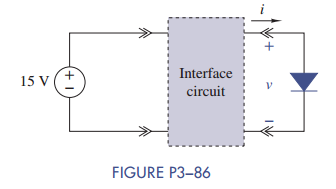### Create an Account

Home / Questions / Figure P3 86 shows an interface circuit connecting a 15-V source to a diode load The i – v...

# Figure P3 86 shows an interface circuit connecting a 15-V source to a diode load The i – v characteristic of the diode is i = 10−14 e40 v 1 a Design an interface circuit so that

Figure P3−86 shows an interface circuit connecting a 15-V source to a diode load. The i – v characteristic of the diode is i = 10−14 (e40 v – 1).

(a) Design an interface circuit so that v = 0:7 V.Subscribe To Get Solution